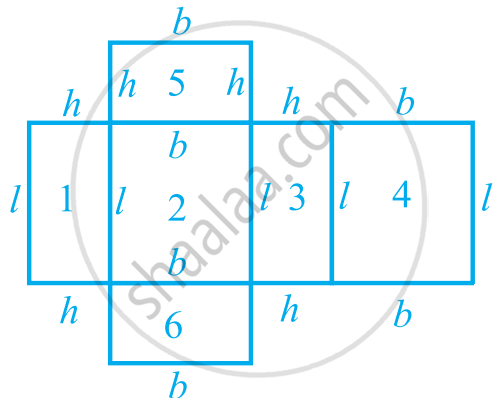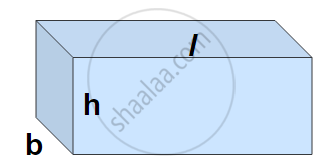# Surface Area of a Cuboid

#### formula

• Cuboid: A cuboid is a convex polyhedron bounded by six quadrilateral faces, whose polyhedral graph is the same as that of a cube. A cuboid looks like a rectangular box. It has 6 faces. Each face has 4 edges. Each face has 4 corners (called vertices).
• Surface of a cuboid: the outer surface of a cuboid is made up of six rectangles (in fact, rectangular regions, called the faces of the cuboid), whose areas can be found by multiplying the length by breadth for each of them separately and then adding the six areas together.
• Lateral surface area of the cuboid: Out of the six faces of a cuboid, we only find the area of the four faces, leaving the bottom and top faces. In such a case, the area of these four faces is called the lateral surface area of the cuboid.

#### formula

• Total surface area of cuboid = 2(lb + bh + lh)
• The lateral surface area of a cuboid = 2h(l + b)

# Cuboid:

A cuboid is a convex polyhedron bounded by six quadrilateral faces, whose polyhedral graph is the same as that of a cube. A cuboid looks like a rectangular box. It has 6 faces. Each face has 4 edges. Each face has 4 corners (called vertices).

# Total Surface Area of a Cuboid:This Figure shows us that the outer surface of a cuboid is made up of six rectangles (in fact, rectangular regions, called the faces of the cuboid), whose areas can be found by multiplying the length by breadth for each of them separately and then adding the six areas together.

Let Consider h = height, b = breadth, l = length of cuboid

The total surface area of a cuboid is equal to the sum of all area of 6 rectangles.

Area of □ MNOP = Area of □ QRST = (l × b) cm2

Area of □ MRSN = Area of □ PQTO = (l × h) cm2

Area of □ MPQR = Area of □ NOTS = (b × h) cm2

Total surface area of cuboid = sum of all area of 6 rectangle = 2(l × b) + 2(b × h) + 2(l × h)

Total surface area of cuboid = 2(lb + bh + lh).

# Lateral Surface area of cuboid:Suppose, out of the six faces of a cuboid, we only find the area of the four faces, leaving the bottom and top faces. In such a case, the area of these four faces is called the lateral surface area of the cuboid.

The lateral surface area of a cuboid is 2h(l + b), i.e., 2 × height × sum of length and breadth.

The lateral surface area of a cuboid = 2h(l + b).

#### Example

How much sheet metal is required to make a closed rectangular box of length 1.5 m, breadth 1.2 m, and height 1.3 m?

length of box = l = 1.5 m,
breadth = b = 1.2 m,
height = h = 1.3 m.

Surface area of box = 2 (l × b + b × h + l × h)

= 2 (1.5 × 1.2 + 1.2 × 1.3 + 1.5 × 1.3)

= 2 (1.80 + 1.56 + 1.95)

= 2 (5.31)

= 10.62 sqm

10.62 sqm of sheet metal will be needed to make the box.

#### Example

An aquarium is in the form of a cuboid whose external measures are 80 cm × 30 cm × 40 cm. The base, side faces and back face are to be covered with a coloured paper. Find the area of the paper needed?
The length of the aquarium = l = 80 cm
Width of the aquarium = b = 30 cm
Height of the aquarium = h = 40 cm
Area of the base = l × b = 80 × 30 = 2400 cm2
Area of the side face = b × h = 30 × 40 = 1200 cm2
Area of the back face = l × h = 80 × 40 = 3200 cm2
Required area = Area of the base + area of the back face + (2 × area of a side face)
= 2400 + 3200 + (2 × 1200) = 8000 cm2
Hence the area of the coloured paper required is 8000 cm2.

#### Example

The internal measures of a cuboidal room are 12 m × 8 m × 4 m. Find the total cost of whitewashing all four walls of a room, if the cost of whitewashing is Rs. 5 per m2. What will be the cost of whitewashing if the ceiling of the room is also whitewashed.
Let the length of the room = l = 12 m
Width of the room = b = 8 m
Height of the room = h = 4 m
Area of the four walls of the room = Perimeter of the base × Height of the room
= 2 (l + b) × h = 2 (12 + 8) × 4
= 2 × 20 × 4 = 160 m2
Cost of white washing per m2 = Rs. 5
Hence the total cost of white washing four walls of the room = Rs. (160 × 5) = Rs. 800.
Area of ceiling is 12 × 8 = 96 m2
Cost of white washing the ceiling = Rs. (96 × 5) = Rs. 480
So the total cost of white washing = Rs. (800 + 480) = Rs. 1280
If you would like to contribute notes or other learning material, please submit them using the button below.

### Shaalaa.com

Total Surface Area & Lateral Surface Area of a Cuboid [00:13:18]
S
0%